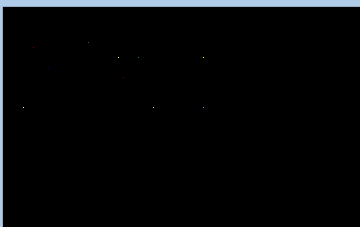# putpixel() function in C

• Difficulty Level : Medium
• Last Updated : 23 Jan, 2018

The header file graphics.h contains putpixel() function which plots a pixel at location (x, y) of specified color.
Syntax :

```void putpixel(int x, int y, int color);

where,
(x, y) is the location at which pixel
is to be put , and color specifies
the color of the pixel.
```

Explanation : A RED color pixel at (50, 40) can be drawn by using putpixel(50, 40, RED). putpixel() function can be used to draw circles, lines and ellipses using various algorithms.

Below is the implementation of putpixel() function.

 `// C Implementation for putpixel()``#include ``#include `` ` `// driver code``int` `main()``{``    ``// gm is Graphics mode which is``    ``// a computer display mode that``    ``// generates image using pixels.``    ``// DETECT is a macro defined in``    ``// "graphics.h" header file``    ``int` `gd = DETECT, gm, color;`` ` `    ``// initgraph initializes the``    ``// graphics system by loading a``    ``// graphics driver from disk``    ``initgraph(&gd, &gm, ``""``);`` ` `    ``// putpixel function``    ``putpixel(85, 35, GREEN);``    ``putpixel(30, 40, RED);``    ``putpixel(115, 50, YELLOW);``    ``putpixel(135, 50, CYAN);``    ``putpixel(45, 60, BLUE);``    ``putpixel(20, 100, WHITE);``    ``putpixel(200, 100, LIGHTBLUE);``    ``putpixel(150, 100, LIGHTGREEN);``    ``putpixel(200, 50, YELLOW);``    ``putpixel(120, 70, RED);`` ` `    ``getch();`` ` `    ``// closegraph function closes the``    ``// graphics mode and deallocates``    ``// all memory allocated by``    ``// graphics system .``    ``closegraph();`` ` `    ``return` `0;``}`

Output :

``````
My Personal Notes arrow_drop_up